# Select Every Other (or Every nth) Row – Excel & Google Sheets

This tutorial will demonstrate how to get a value from every other (or every nth) row in Excel and Google Sheets.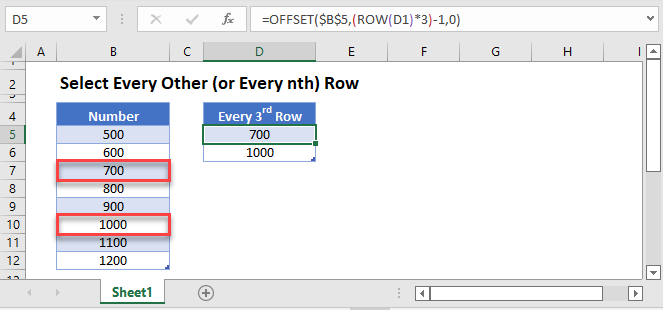## Identify Every Other Row

To identify every other row, we can use the MOD function to let us know if each Row is divisible by a certain number. Here we will use 2 to identify every other row (odd / even).

``=MOD(ROW(B3),2)``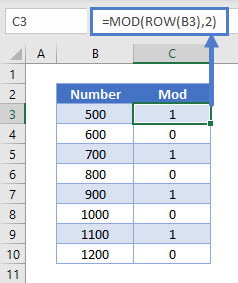We will walkthrough this below.

### ROW Function – Return Row number

First, we will use the ROW Function to tell us which row we are in:

``=ROW(B3)``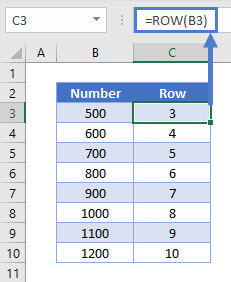In the next section, we will use the MOD function to let us know if the row number is divisible by a certain number, in this case – 2.

### MOD Function – Show if the Row is Divisible by 2

The MOD Function will show us if the row that we are in is divisible by 2.

``=MOD(C3,2)``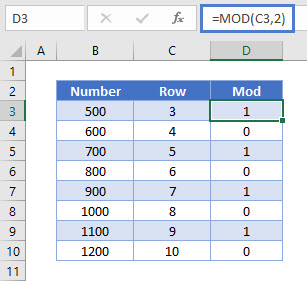Combining these functions yields the original formula.

``=MOD(ROW(B3),2)``

## Select Every nth Row

To get the every 3rd (nth) row, we change the number to divide by to 3 (n).

``=MOD(c3,3)``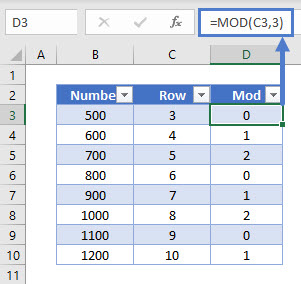We can switch the filter on to filter on the MOD result required to show specific rows.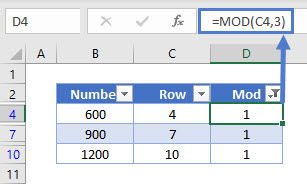## Get Value from Every nth Row

To get the value from every other row or nth row, we can use the OFFSET and ROW functions.

``=OFFSET(\$B\$3,(ROW(D1)*2)-1,0)``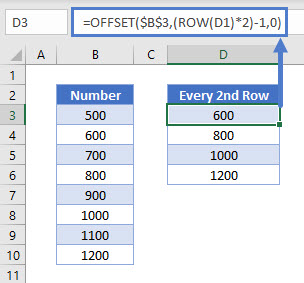We will walkthrough this below.

## Return the nth Row

First, we will use the ROW Function to pick up the 1st Row. Copying this formula down, will mean that the Row number picked up will dynamically change as the formula does.

``=ROW(D1)``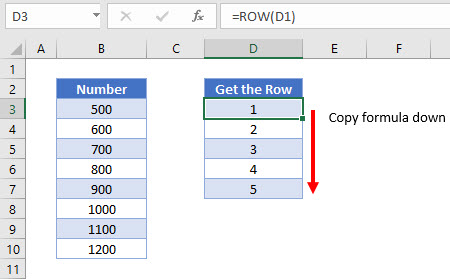In the next section, we will use the OFFSET function to get the value from the Row multiplied by the value you wish to offset the row by.

``=OFFSET(\$B\$3,(D3*2)-1,0)``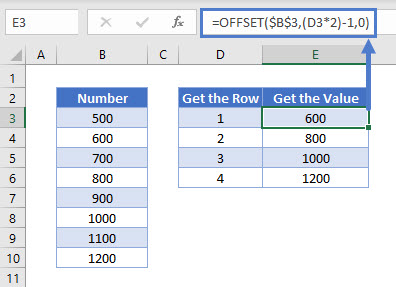Combining these functions yields the original formula.

``=OFFSET(\$B\$3,(ROW(E1)*2)-1,0)``

### Easy Excel Automation## Write VBA Code

We can use a VBA procedure to loop through a range of cells and return the value from every 2nd or nth cell.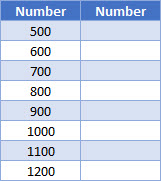The following procedure will loop through the range of cells and populate every second row in Column C with the corresponding number from column B.

``````Sub SelectAltRows()
Dim rng1 As Range
Dim rng2 As Range
Dim x As Integer
Dim NoRws As Integer
'select the range
Set rng1 = Range("B1:B10")
'count the rows
NoRws = rng1.Rows.Count
'loop through every second cell of the range
For x = 1 To NoRws Step 2
'put the value from column B into column C
rng1.Cells(x, 1).Offset(0, 1) = rng1.Cells(x, 1)
Next
End Sub
``````

The result of running this procedure would be: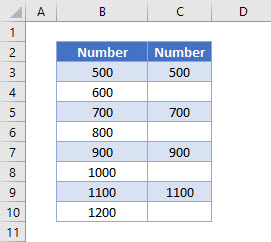## Select Every Other (or Every nth) Row in Google Sheets

These formulas work exactly the same in Google Sheets as in Excel.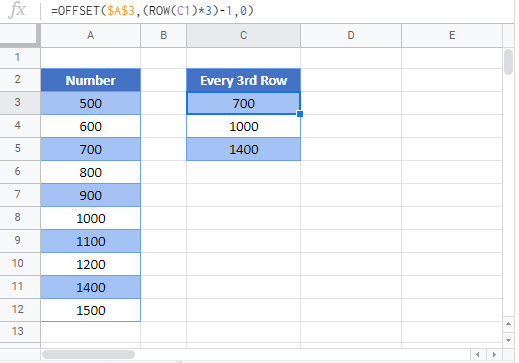### Excel Practice Worksheet

Practice Excel functions and formulas with our 100% free practice worksheets!